Tamilnadu Samacheer Kalvi 9th Maths Solutions Chapter 4 Geometry Ex 4.6

9th Maths Geometry Exercise 4.6 Question 1.
Draw a triangle ABC, where AB = 8 cm, BC = 6 cm and ∠B = 70° and locate its circumcentre and draw the circumcircle.
Solution:
∆ABC, where AB = 8 cm,
BC = 6 cm,
B = 70°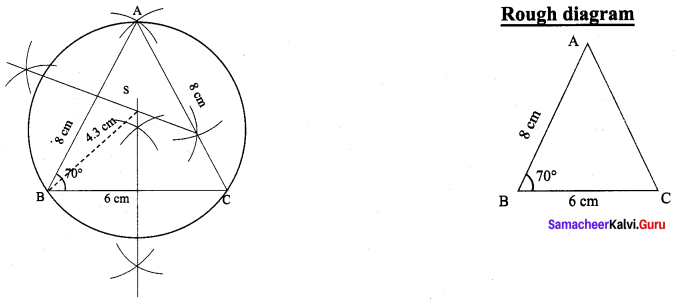Construction:
(i) Draw the ∆ABC with the given measurements.
(ii) Construct the perpendicular bisector at any two sides (AB and BC) and let them meet at S which is the circumcircle.
(iii) S as centre and SA = SB = SC as radius, draw the circumcircle to pass through A, B, and C. Circum radius = 4.3 cm.

9th Maths Exercise 4.6 Question 2.
Construct the right triangle PQR whose perpendicular sides are 4.5 cm and 6 cm. Also locate its circumcentre and draw the circumcircle.
Solution:
Right triangle PQR whose perpendicular sides are 4.5 cm and 6 cm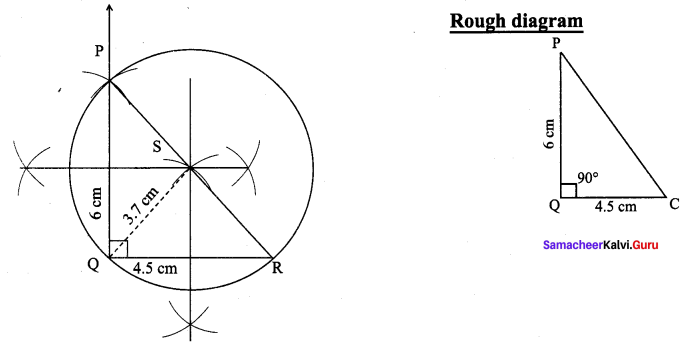Construction :
(i) Draw the right triangle PQR with the given measurements.
(ii) Construct the perpendicular bisector at any two sides (PQ and QR) and let them meet at S which is the circumcentre.
(iii) S as centre and SP = SQ = SR as radius, draw the circumcircle to pass through P, Q and R. Circumradius = 3.7 cm.

9th Maths Geometry Exercise 4.6 In Tamil Question 3.
Construct ∆ABC with AB = 5 cm ∠B = 100° and BC = 6 cm. Also locate its circumcentre draw circumcircle.
Solution: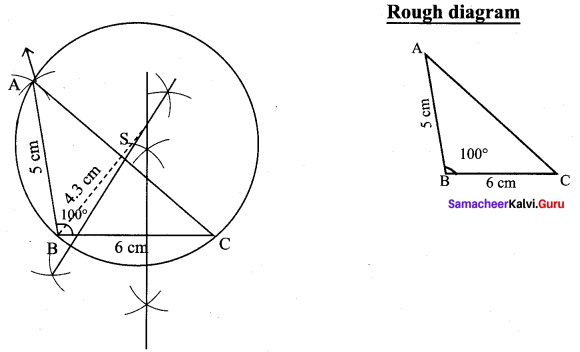Construction :
(i) Draw the ∆ABC with the given measurements.
(ii) Construct the perpendicular bisector at any two sides (BC and AC) and let them meet at S which is the circumcentre.
(iii) S as centre and SA = SB = SC as radius, draw the circumcircle to pass through A, B, and C. Circumradius = 4.3 cm.

Ex 4.6 Class 9 Maths Question 4.
Construct an isosceles triangle PQR where PQ = PR and ∠Q = 50°, QR = 7cm. Also draw its circumcircle.
Solution:
Isosceles triangle PQR where PQ = PR and Q = 50°, QR = 7 cm.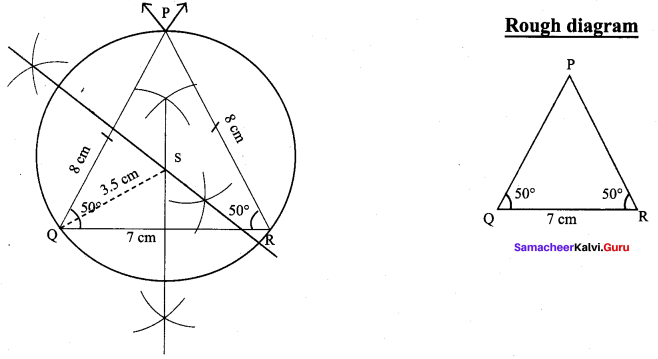Construction :
(i) Draw the ∆PQR with the given measurements.
(ii) Construct the perpendicular bisector at any two sides (PQ and QR) and let them meet at S which is the circumcentre.
(iii) S as centre and SP = SQ = SR as radius, draw the circumcircle to pass through P, Q, R. Circumradius = 3.5 cm.

9th Standard Maths Exercise 4.6 Question 5.
Draw an equilateral triangle of sides 6.5 cm and locate its incentre. Also draw the incircle.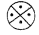Solution:
Side = 6.5 cm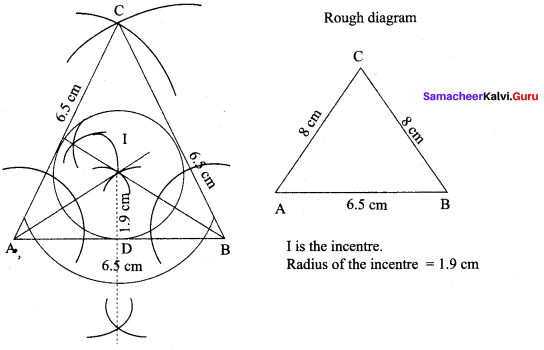Construction :
Step 1 : Draw ∆ABC with AB = BC = CA = 6.5 cm
Step 2 : Construct angle bisectors of any two angles (A and B) and let them meet at I.I is the incentre of ∆ABC.
Step 3 : Draw perpendicular from I to any one of the side (AB) to meet AB at D.
Step 4 : With I as centre, ID as radius draw the circle. This circle touches all the sides of triangle internally.

Exercise 4.6 Class 9 Maths Question 6.
Draw a right triangle whose hypotenuse is 10 cm and one of the legs is 8 cm. Locate its incentre and also draw the incircle.
Solution:
hypotenuse = 10 cm
One of the legs = 8 cm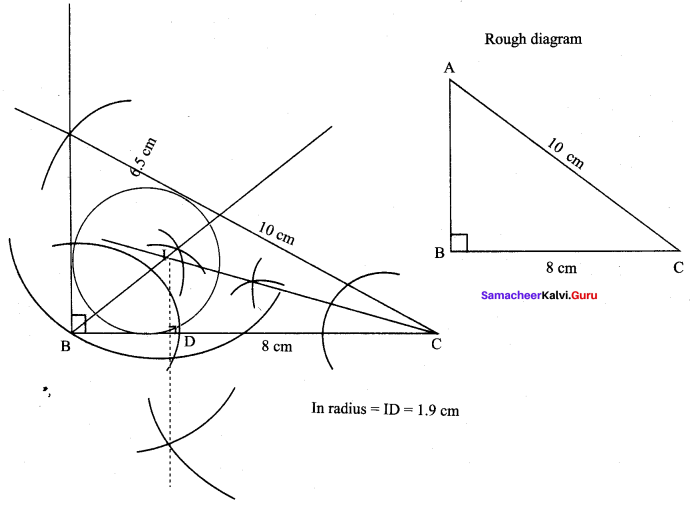Step 1 : Draw AABC with BC = 8 cm. AC = 10 cm with right angle at B.
Step 2 : Construct angle bisectors of any two angles (B and C) and let them meet at 1.1 is the incentre.
Step 3 : Draw perpendicular from I to any side of the triangle to meet BC at D.
Step 4 : With I as centre, ID as radius draw the incircle, which touches all the three sides of the triangle internally. In radius = 1.9 cm.

9th Maths Geometry Exercise 4.6 Solutions Question 7.
Draw ∆ABC given AB = 9 cm, ∠CAB = 115° and ∆ABC = 40°. Locate its incentre and also draw the incircle. (Note: You can check from the above examples that the incentre of any triangle is always in its interior).
Solution: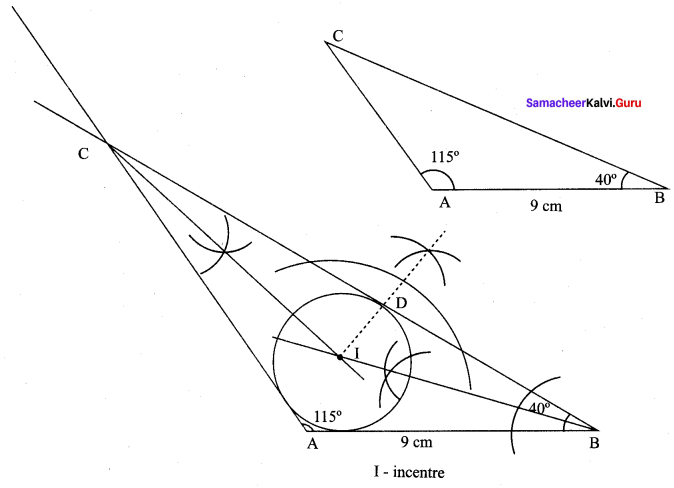Construction :
Step 1 : Draw ∆ABC with AB = 9 cm. ∠A = 115°,∠B = 40°.
Step 2 : Construct angle bisectors of any two angles (B and C). Let them meet at I.I is the incentre of ∆ABC.
Step 3 : Draw perpendicular from I to any side (BC) to meet BC at D.
Step 4 : Draw incircle, with I as centre and ID a radius. Measures the in radius.

9th Geometry 4.6 Question 8.
Construct ∆ABC in which AB = BC = 6 cm and B = 80° . Locate its incentre and draw the incircle.
Solution:
In ∆ABC, AB = BC = 6 cm, ∠B = 80°.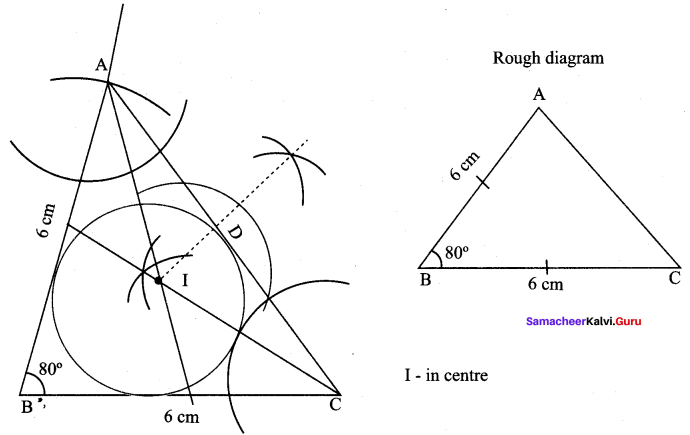Construction :
Step 1 : Draw AABC with BC = 6 cm. AB = 6 cm, AB = 6 cm, and ∠B = 80°.
Step 2 : Construct the incentre I and ID is the in radius, as in the previous sums.
Step 3 : Draw incircle with I as centre and ID as radius. It touches all the three sides internally.